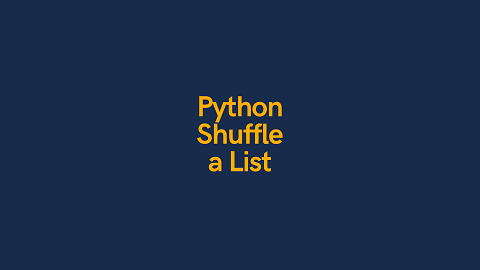# How to Shuffle List of Objects in Python

Often python developers employ lists and objects to store data. Sometimes people even create a list of objects for that matter. Sometimes you may need to shuffle list of objects in Python. There are several simple ways to do this. In this article, we will learn how to shuffle list of objects in Python.

## How to Shuffle List of Objects in Python

We will learn a couple of ways to shuffle list of objects in Python.

## 1. Using Random

Random is a built in python library that offers different functions to introduce randomness in your application or script. It can be used to generate random number or shuffle list of items. Here is a simple code to shuffle list of objects in Python using random library.

```from random import shuffle

x = [[i] for i in range(10)]
shuffle(x)
print(x)```

In the above code, we first create a list of lists using list comprehension and store it in variable x. Then we simply call the function shuffle() on this list. It returns None and shuffles the list in place, meaning it will modify the original list instead of creating a separate list of shuffled items.

Please note, you can also use this method on a list of plain integers also, not just a list of objects.

```from random import shuffle

x = [i for i in range(10)] #[0, 1, 2, 3, 4, 5, 6, 7, 8, 9]
shuffle(x)
print(x) # [9, 0, 5, 4, 2, 8, 3, 7, 6, 1]```

## 2. Using Numpy

You can also use Numpy library to shuffle a list of items. Numpy is a popular library used for financial and scientific tasks in Python.

```import numpy as np
b = np.arange(10)
np.random.shuffle(b)
print(b)```

In the above code, we create a list of items using np.arange() function. Then we call np.random.shuffle() function. This function also shuffles the list of items in place.

This function can also be used on a list of integers, not just list of objects.

In this article, we have learnt a couple of simple ways to shuffle list of objects in Python.